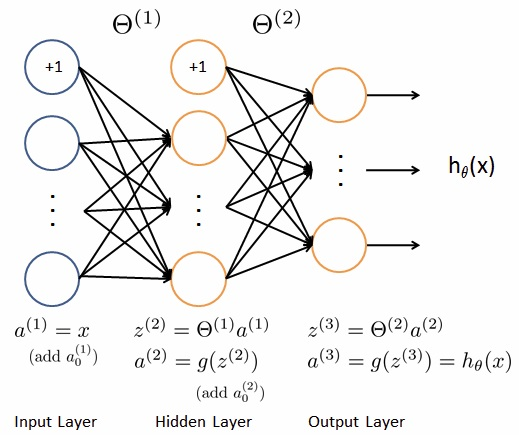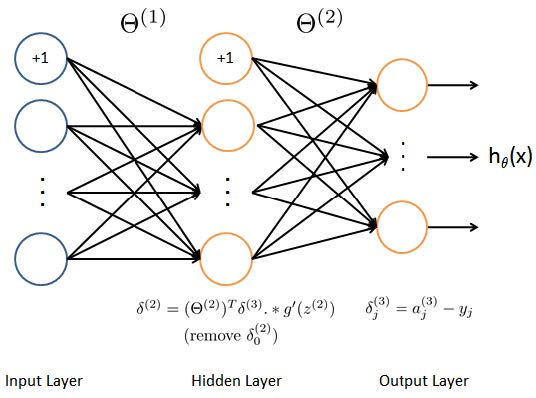Neural Networks Learning

## Cost Fuction1. For a neural network like the pic above, the cost function should be like:
$$J(\theta) = \frac {1}{m} \sum\limits_{i=1}^{m}\sum\limits_{k=1}^{K} [-y_k^{(i)} log(h_\theta (x^{(i)})_k) - (1 - y_k^{(i)}) log(1 - h_\theta (x^{(i)})_k)]$$
2. In which there are m training data and the output layer has K units. The cost function will sum all the outputs from all the data.
3. Similarly, the regularization term just sum up the square of all the parameters from all the layers:
$$\frac {\lambda}{2m} \sum\limits_{j=1}^{s^{(l)}} \sum\limits_{k=2}^{s^{(l+1)}} \sum\limits_{l=1}^{n} [(\Theta_{jk}^{(l)})^2]$$
4. Note that you should not be regularizing the terms that correspond to the bias. For the matrices here, this corresponds to the first column of each matrix, so k should start from index 2.

## Backpropagation Algorithm1. In order to use optimization algorithms like gradient descent, we must calculate the derivative of the cost function. First we should calculate $\delta$ for each layer using backpropagation algorithm. From $layer_l$ to $layer_{l+1}$, we have:
$$\delta^{(output)} = a^{(output)} - y \\ \delta^{(l)} = [\Theta^{(l)}]^T \delta^{(l+1)} .* g’(z^{(l)})$$
2. For sigmoid function, $g’(z) = g(z)(1-g(z))$
3. Then we have the derivative:
$$\frac {\partial}{\partial \Theta_{ij}^{(l)}} J(\Theta) = a_j^{(l)} \delta_i^{(l+1)} \\ \Delta^{(l)} = \delta^{(l+1)} (a^{(l)})^T$$
4. Instead of calculate the $\Delta$ for all the training data, we could sum up all the inputs independantly, like:
\begin{align} & \text{From i = 1 to m, do:} \\ && \Delta^{(l)} += \delta^{(l+1)} (a^{(l)})^T \\ & \text{Then we have:} \\ && \frac {\partial}{\partial \Theta_{ij}^{(l)}} J(\Theta) = \frac {1}{m} \Delta_{ij}^{(l)} \end{align}
• (This step is the main step for mapreduce, see Large Scale Machine Learning)
5. Then add regularzation term for partial derivative, we have:
$$\frac {\partial}{\partial \Theta_{ij}^{(l)}} J(\Theta) = \frac {1}{m} \Delta_{ij}^{(l)} + \frac {\lambda}{m} \Theta_{ij}^{(l)}$$
6. Finally, using $J(\Theta)$ and $\frac {\partial}{\partial \Theta_{ij}^{(l)}} J(\Theta)$, we could minimize the cost function using gradient descent as well as other optimization algorithms.

## Optional Section: How Backpropagation Works

Using the same model as the last section:
\begin{align} \frac {\partial J}{\partial \Theta_{ij}^{(1)}} & = \frac {\partial J}{\partial a_i^{(2)}} \frac {\partial a_i^{(2)}}{\partial z_i^{(2)}} \frac {\partial z_i^{(2)}}{\partial \Theta_{ij}^{(1)}} \\ & = \frac {\partial J}{\partial a_i^{(2)}} g’(z_i^{(2)}) a_i^{(1)} \end{align}
Then taking the total derivative with respect to $z^{(3)}$, a recursive expression for the derivative is obtained:
\begin{align} \frac {\partial J}{\partial a_i^{(2)}} & = \sum\limits_{m=1}^{s_3} \frac {\partial J}{\partial z_m^{(3)}} \frac {\partial z_m^{(3)}}{\partial a_i^{(2)}} \\ & = \sum\limits_{m=1}^{s_3} \frac {\partial J}{\partial a_m^{(3)}} \frac {\partial a_m^{(3)}}{\partial z_m^{(3)}} \frac {\partial z_m^{(3)}}{\partial a_i^{(2)}} \\ & = \sum\limits_{m=1}^{s_3} \frac {\partial J}{\partial a_m^{(3)}} g’(z_m^{(3)}) \Theta_{mi}^{(2)} \end{align}

Let $\delta_i^{(2)} = \frac {\partial J}{\partial a_i^{(2)}} g’(z_i^{(2)})$, then from the result of the first formula We have: $\frac {\partial J}{\partial \Theta_{ij}^{(1)}} = \delta_i^{(2)} a_i^{(1)}$, in which recursively, $\delta_i^{(2)} = (\sum\limits_{m=1}^{s_3} \delta_m^{(3)}\Theta_{mi}^{(2)}) g’(z_i^{(2)})$